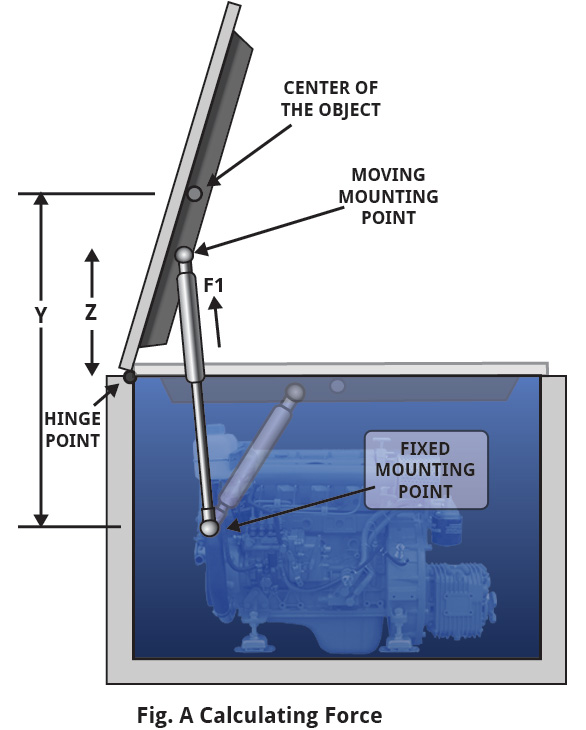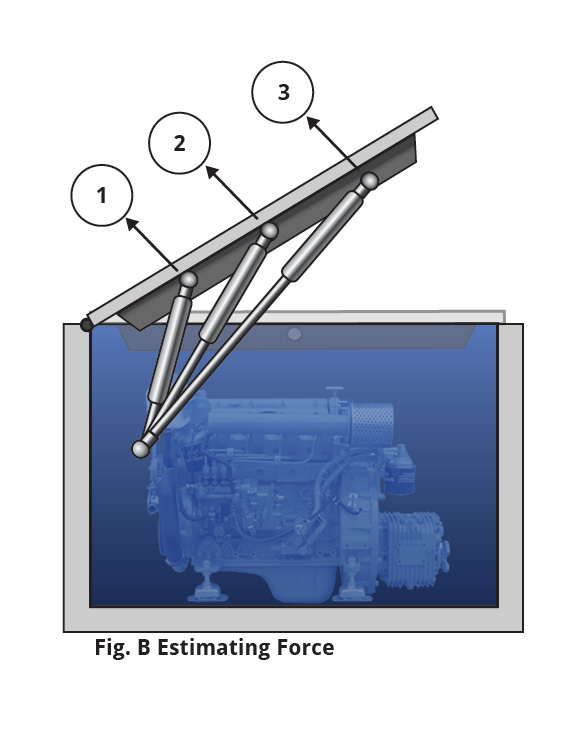Gas Springs

Choosing the right force – metric

Gas Springs are typically used for assisting in lifting a lid as well as holding it open, similar to how a hatchback on a car operates. They are also used on access covers, doors, windows, conveyors, adjustable table and seats, and more.

All gas springs contain pressurized nitrogen gas as well as hydraulic fluid that lubricates the seal and provides slam-resistant dampening at the end of the stroke. You should store and install gas springs rod-end down for longer service life.

If you need a gas spring but you don’t know the force needed, the most accurate way to determine that force is to calculate it using the formula below.

Calculating force

Use the following formula to determine the approximate force you will need for your application.Calculator

FORCE = (W x Y) / (Z x N)

W = Weight of object to be moved.

Y = Distance from fixed mounting point to center of the object (mm).

Z = Distance from hinge point to moving mounting point (mm).

N = Number of springs.

If you do not have all of the necessary information to use the above formula, you can use our estimated force calculation. With either method of determining force, you will need to know the weight of the object you are moving.

Estemating force

The force changes according to where you want to install the gas spring. In Fig. B several installations of the gas springs are shown. For the estimated force information of these gas spring installations please see thd example below.Example:

If weight of lid = 250N

1= Use a 500N force gas spring

2= Use a 250N force gas spring

3= Use a 125N force gas spring

*NOTE: If you use two gas springs, divide the force in half.

The optimal position for a gas spring is usually halfway between the hinge and the center of the object you are lifting (force should be approx. twice the weight of the lid). You can use a shorter spring to reduce the chance of the spring obstructing access.

Gas springs can lose pressure over time, so it is best to choose a force approximately 20% greater than needed.